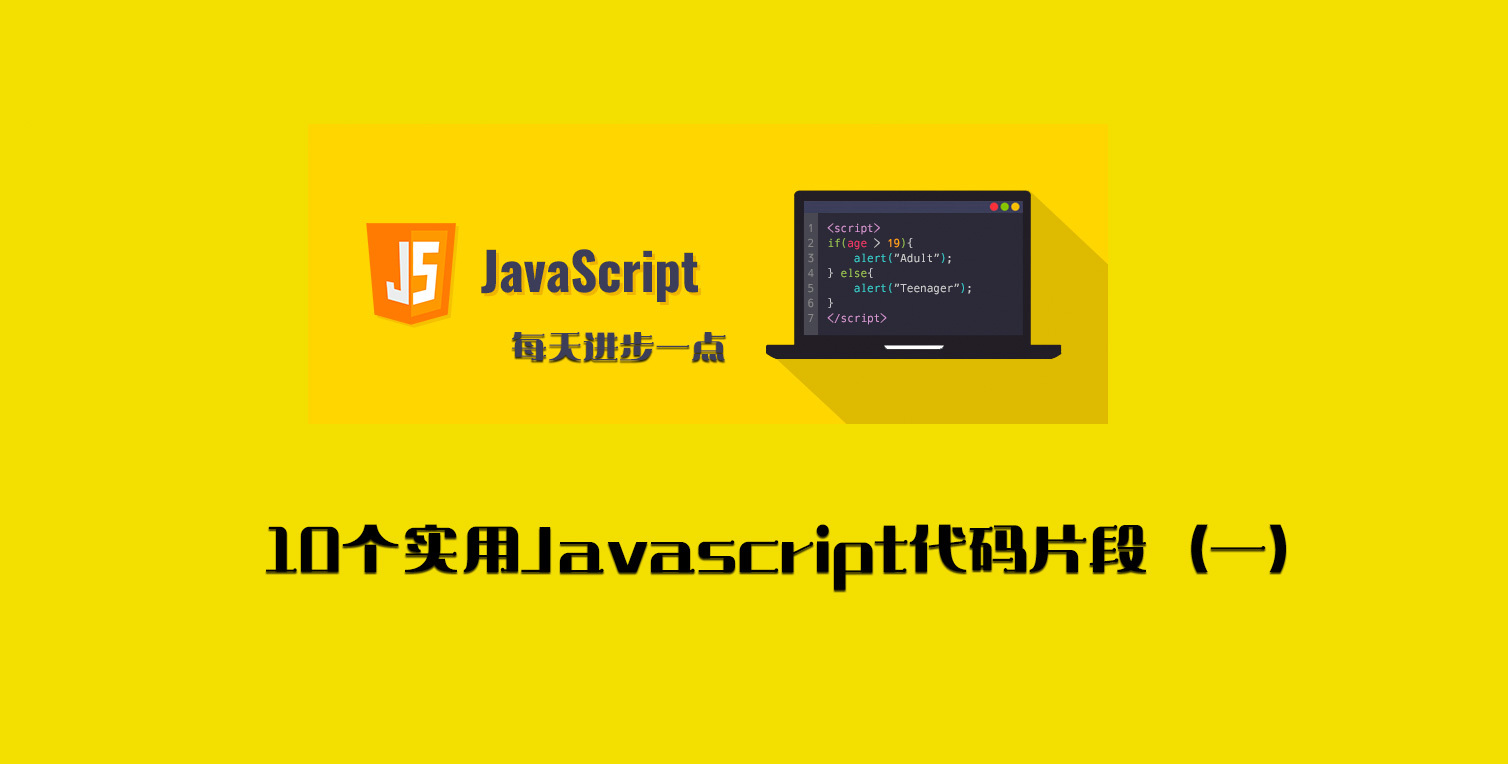# 每天学习 10 个实用 Javascript 代码片段（一）devpoint### 1. localStorage

localStorage 是 HTML5 中的本地持久化存储方法之一，也是前端项目常用的本地存储方案之一。localStorage 存储的数据只要用户不去主动清除是永久存储的，存储的值只能是 string 类型，不能跨浏览器，不能跨域名访问，存储大小一般是 5M 左右。下面的代码片段是 localStorage 数据的存储、获取、清除。

### 6. arrayToHtmlList

const arrayToHtmlList = (array, tag = "li") =>    array.map((item) => <${tag}>${item}</\${tag}>).join("");console.log(arrayToHtmlList(["第一条", "第二条"])); // <li>第一条</li><li>第二条</li>console.log(arrayToHtmlList(["第一条", "第二条"], "p")); // <p>第一条</p><p>第二条</p>

### 7. allEqual

const allEqual = (array) => array.every((val) => val === array);console.log(allEqual([1, 1, 1, "1"])); // falseconsole.log(allEqual([1, 1, 1, 2])); // falseconsole.log(allEqual([1, 1, 1, 1])); // trueconsole.log(allEqual(["a", "a", "a"])); // true

### 8. countOccurrences

const countOccurrences = (array, value) =>    array.reduce(        (accumulator, current) =>            current === value ? accumulator + 1 : accumulator,        0    );console.log(countOccurrences([..."chinese"], "e")); // 2console.log(countOccurrences([1, 3, 3, 4, 3, 3, 2, 3], 3)); // 5

### 9. capitalizeWord

const capitalizeWord = (string) =>    string.replace(/\b[a-z]/g, (char) => char.toUpperCase());console.log(capitalizeWord("hello world in javascript")); // Hello World In Javascript

### 10. byteSize

const byteSize = (string) => new Blob([string]).size;console.log(byteSize("hello")); // 5console.log(byteSize("中国")); // 6## 评论